# React Hooks 心智负担：消个抖都消不利索了React Hooks已经有三年多的时间了，团队中也基本上已经很少看到class组件的身影，也很少再看到用不用hooks的争论，笔者认为，hooks优势确实明显，但是随之而来的心智负担也确实存在。就比如，按钮消个抖，非常常见的业务诉求，但是实际写起来，确实并不容易。

## 业务背景

``````import React, { useCallback, useState } from 'react';

const fakeFetch = (v) => {
return new Promise((resolve) => {
setTimeout(() => {
resolve(JSON.stringify(v));
}, 500);
});
};

export default function App() {
const [value, setValue] = useState('');
const [res, setRes] = useState('');
const [fakeFetchCount, setFakeFetchCount] = useState(0);

const getRes = useCallback((v) => {
setFakeFetchCount((pre) => pre + 1);
fakeFetch({
value: v,
}).then((res) => setRes(res));
}, []);

const onChange = useCallback((e) => {
setValue(e.target.value);
getRes(e.target.value);
}, []);

return (
<div>
<input value={value} onChange={onChange} />
<div>{res}</div>
<div>fetch次数: {fakeFetchCount}</div>
</div>
);
}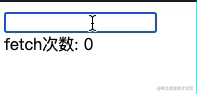## 消抖方法

### 传统的消抖

``````function debounce(func, waitTime) {
let timer = null;

return function (...args) {
if (timer) {
clearTimeout(timer);
}
timer = setTimeout(() => {
func.apply(this, args);
}, waitTime);
};
}

### hooks 写法

#### 直接消抖

``````// version 1： 直接消抖的代码
import React, { useCallback, useState, useEffect } from 'react';

function debounce(func, waitTime) {
let timer = null;

return function (...args) {
if (timer) {
clearTimeout(timer);
}
timer = setTimeout(() => {
func.apply(this, args);
}, waitTime);
};
}

const fakeFetch = (v) => {
return new Promise((resolve) => {
setTimeout(() => {
resolve(JSON.stringify(v));
}, 500);
});
};

export default function App() {
const [value, setValue] = useState('');
const [res, setRes] = useState('');
const [fakeFetchCount, setFakeFetchCount] = useState(0);

// 用debounce函数包裹原函数
const getRes = useCallback(
debounce((v) => {
setFakeFetchCount((pre) => pre + 1);
fakeFetch({
value: v,
}).then((res) => {
setRes(res);
});
}, 500),
[]
);

const onChange = useCallback(
(e) => {
setValue(e.target.value);
getRes(e.target.value);
},
[getRes]
);

return (
<div>
<input value={value} onChange={onChange} />
<div>{res}</div>
<div>fetch次数: {fakeFetchCount}</div>
</div>
);
}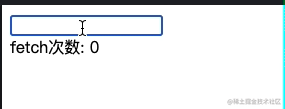``````const getRes = useCallback(
debounce((v) => {
setFakeFetchCount((pre) => pre + 1);
fakeFetch({
value: v,
}).then((res) => {
setRes(res);
});
}, 500),
[]
);

#### 加入了依赖项

``````// version2: 加入了停留时长依赖的消抖
import React, { useCallback, useState, useEffect } from 'react';

function debounce(func, waitTime) {
let timer = null;

return function (...args) {
if (timer) {
clearTimeout(timer);
}
timer = setTimeout(() => {
func.apply(this, args);
}, waitTime);
};
}

const fakeFetch = (v) => {
return new Promise((resolve) => {
setTimeout(() => {
resolve(JSON.stringify(v));
}, 500);
});
};

export default function App() {
const [value, setValue] = useState('');
const [res, setRes] = useState('');
const [fakeFetchCount, setFakeFetchCount] = useState(0);
// 增加用户停留时间状态
const [duration, setDuration] = useState(0);

// 用户停留时长更新
useEffect(() => {
setInterval(() => {
setDuration((pre) => pre + 1);
}, 1000);
}, []);

const getRes = useCallback(
debounce((v) => {
setFakeFetchCount((pre) => pre + 1);
fakeFetch({
value: v,
// 请求时携带duration参数
duration,
}).then((res) => {
setRes(res);
});
}, 500),
// 增加对用户停留时长状态的依赖
[duration]
);

const onChange = useCallback(
(e) => {
setValue(e.target.value);
getRes(e.target.value);
},
[getRes]
);

return (
<div>
<input value={value} onChange={onChange} />
<div>{res}</div>
<div>fetch次数: {fakeFetchCount}</div>
</div>
);
}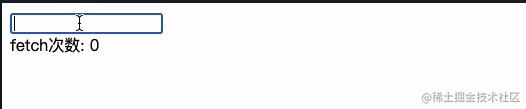#### 维持同一个 timer

debounce 函数在每次组件渲染后，都会新生成一个函数，此时如果 useCallback 中有依赖，那么上一次的消抖函数生成的定时器可能就没有清理，导致依旧执行带来预期不一致。

``````// version 4： 维持同一个 timer
import React, { useCallback, useState, useEffect, useRef } from 'react';

const fakeFetch = (v) => {
return new Promise((resolve) => {
setTimeout(() => {
resolve(JSON.stringify(v));
}, 500);
});
};

export default function App() {
const [value, setValue] = useState('');
const [res, setRes] = useState('');
const [fakeFetchCount, setFakeFetchCount] = useState(0);
const [duration, setDuration] = useState(0);

useEffect(() => {
setInterval(() => {
setDuration((pre) => pre + 1);
}, 1000);
}, []);

// 用 ref 维持同一个timer
const timerRef = useRef(null);

const getRes = useCallback(
(v) => {
if (timerRef.current) {
clearTimeout(timerRef.current);
}
timerRef.current = setTimeout(() => {
setFakeFetchCount((pre) => pre + 1);
fakeFetch({
value: v,
duration,
}).then((res) => {
setRes(res);
});
}, 500);
},
[duration]
);

const onChange = useCallback(
(e) => {
setValue(e.target.value);
getRes(e.target.value);
},
[getRes]
);

return (
<div>
<input value={value} onChange={onChange} />
<div>{res}</div>
<div>停留时长：{duration}s</div>
<div>fetch次数: {fakeFetchCount}</div>
</div>
);
}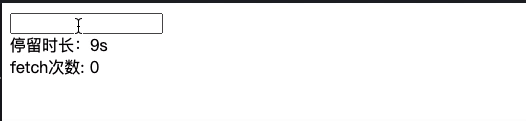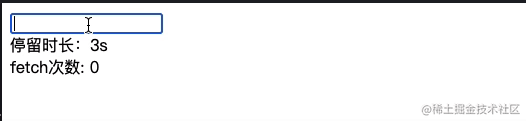#### 维持同一个函数

``````// version5: 维持同一个timer, func
import React, { useCallback, useState, useEffect, useRef } from 'react';

const fakeFetch = (v) => {
return new Promise((resolve) => {
setTimeout(() => {
resolve(JSON.stringify(v));
}, 500);
});
};

export default function App() {
const [value, setValue] = useState('');
const [res, setRes] = useState('');
const [fakeFetchCount, setFakeFetchCount] = useState(0);
const [duration, setDuration] = useState(0);

useEffect(() => {
setInterval(() => {
setDuration((pre) => pre + 1);
}, 1000);
}, []);

const timerRef = useRef(null);

// ref 维持函数
const funcRef = useRef(null);

// 原函数定义
const getRes = useCallback(
(v) => {
setFakeFetchCount((pre) => pre + 1);
fakeFetch({
value: v,
duration,
}).then((res) => {
setRes(res);
});
},
[duration]
);

// 始终跟原函数保持一致
funcRef.current = getRes;

// 消抖逻辑
const getResDebounced = useCallback((...args) => {
if (timerRef.current) {
clearTimeout(timerRef.current);
}
timerRef.current = setTimeout(() => {
// 定时器中通过访问ref，避免闭包带来的访问旧值的问题
funcRef.current.apply(null, args);
}, 5000);
}, []);

const onChange = useCallback(
(e) => {
setValue(e.target.value);
getResDebounced(e.target.value);
},
[getResDebounced]
);

return (
<div>
<input value={value} onChange={onChange} />
<div>{res}</div>
<div>停留时长：{duration}s</div>
<div>fetch次数: {fakeFetchCount}</div>
</div>
);
}

1. 新建funcRef，同步 getRes 函数，始终保持最新
2. 新建 getRefDebounced 函数，该函数中使用 funcRef.current 作为定时器中执行的函数。

#### 封装自定义hook

``````function useDebounceFn(fn, delay) {
const timerRef = useRef(null);
const fnRef = useRef(fn);
fnRef.current = fn;

const fnDebounced = useCallback(
function (...args) {
if (timerRef.current) {
clearTimeout(timerRef.current);
}
timerRef.current = setTimeout(() => {
fnRef.current.apply(this, args);
}, delay);
},
[delay]
);

return fnDebounced;
}

1. fnRef.current = fn, 要不要用 useEffect 包裹，这里我的观点是不需要，useEffect会做一次比较，其实有比较的这个功夫，赋值也不耽误事，实际套个循环（hooks并不推荐，此处仅做实验），1000次左右的话，直接赋值会在0.1ms左右，而useEffect会在1ms左右，差别也不算大。套不套 useEffect 影响真的不大。

2. fnDebounced中的 useCallback 中的函数，一定要使用 普通函数 而非 箭头函数。因为一旦使用箭头函数，那么 this 其实外面就改变不了了。虽然在函数组件中，this 变得越来越不常用，但是可以通过普通函数保留这个 this， 以便外界需要的时候自行使用。

3. 依赖项 deps 放在哪里？不需要放 deps 的地方，全部交由外部传递进来的fn处理，如果需要根据 deps 变化而更新消抖函数，那么直接在外部的 fn 中处理即可。

``````// version5: 维持同一个timer, func
import React, { useCallback, useState, useEffect, useRef } from 'react';

const fakeFetch = (v) => {
return new Promise((resolve) => {
setTimeout(() => {
resolve(JSON.stringify(v));
}, 500);
});
};

function useDebounceFn(fn, delay) {
const timerRef = useRef(null);
const fnRef = useRef(fn);

fnRef.current = fn;

const fnDebounced = useCallback(
function (...args) {
if (timerRef.current) {
clearTimeout(timerRef.current);
}
timerRef.current = setTimeout(() => {
fnRef.current.apply(this, args);
}, delay);
},
[delay]
);

return fnDebounced;
}

export default function App() {
const [value, setValue] = useState('');
const [res, setRes] = useState('');
const [fakeFetchCount, setFakeFetchCount] = useState(0);
const [duration, setDuration] = useState(0);

useEffect(() => {
setInterval(() => {
setDuration((pre) => pre + 1);
}, 1000);
}, []);

const getRes = useCallback(
(v) => {
setFakeFetchCount((pre) => pre + 1);
fakeFetch({
value: v,
duration,
}).then((res) => {
setRes(res);
});
},
[duration]
);

const getResDebounced = useDebounceFn(getRes, 500);

const onChange = useCallback(
(e) => {
setValue(e.target.value);
getResDebounced(e.target.value);
},
[getResDebounced]
);

return (
<div>
<input value={value} onChange={onChange} />
<div>{res}</div>
<div>停留时长：{duration}s</div>
<div>fetch次数: {fakeFetchCount}</div>
</div>
);
}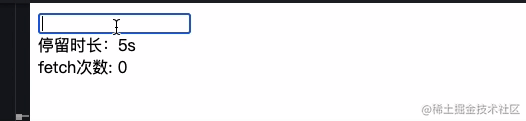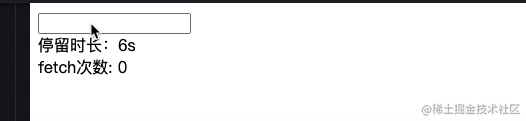``````function useDebounceFn(fn, delay) {
const timerRef = useRef(null);
const fnRef = useRef(fn);

fnRef.current = fn;

// 增加组件卸载时，timer 的清除
useEffect(() => {
return () => {
if (timerRef.current) {
clearTimeout(timerRef.current);
}
};
}, []);

const fnDebounced = useCallback(
function (...args) {
if (timerRef.current) {
clearTimeout(timerRef.current);
}
timerRef.current = setTimeout(() => {
fnRef.current.apply(this, args);
}, delay);
},
[delay]
);

return fnDebounced;
}